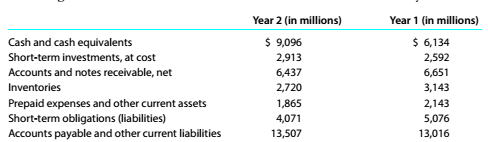Chapter 9, Problem 9.7E

Chapter
Section
Textbook Problem

Current position analysis PepsiCo. Inc. (PEP), the parent company of Frito-Lay™ snack foods and Pepsi beverages, had the following current assets and current liabilities at the end of two recent years:a.Determine the (1) current ratio and (2) quick ratio for both years. Round to one decimal place. b. What conclusions can you draw from these data?

To determine

Concept Introduction:

Current Ratio: Current ratio is the ratio of current assets with current liabilities it tells about the capability of a company to meet its daily expenses. Best current ratio is 2:1.

Quick Ratio: Quick ratio is the ratio of quick assets with current liabilities, it tells about the liquidity of the company/ business. Best possible quick ratio is 1:1.

Requirement-1:

To Calculate:

Current ratio and quick ratio.

Explanation
 Particulars Year 1 Year 2 Current Assets(A) 23031 20663 Current Liabilities (B) 17578 18092 Inventory (C) 2720 3143
To determine

Concept Introduction:

Current Ratio: Current ratio is the ratio of current assets with current liabilities it tells about the how much company strong to meet its day to day expenses. Best current ratio is 2:1.

Quick Ratio: Quick ratio is the ratio of quick assets with current liabilities it tells about the liquidity of the company/ business. Best possible quick ratio is 1:1.

Requirement-2:

To Indicate:

What Conclusion from above ratios?

Still sussing out bartleby?

Check out a sample textbook solution.

See a sample solution

The Solution to Your Study Problems

Bartleby provides explanations to thousands of textbook problems written by our experts, many with advanced degrees!

Get Started

An independent contractor is one who works under the control and direction of an employer.

College Accounting, Chapters 1-27 (New in Accounting from Heintz and Parry)

Calculate the missing items in the following:

College Accounting (Book Only): A Career Approach

INTEREST RATE PREMIUMS A 5-year Treasury bond has a 5.2% yield. A 10-year Treasury bond yields 6.4%, and a 10-y...

Fundamentals of Financial Management, Concise Edition (with Thomson ONE - Business School Edition, 1 term (6 months) Printed Access Card) (MindTap Course List)